# Preface

In this section, we analyze differential equation modeling Duffing oscillator, named after Georg Duffing (1861--1944). It provides an example of a periodically forced oscillator with a nonlinear elasticity. The Duffing equation is an example of a dynamical system that exhibits chaotic behavior. For this type of system, there are frequencies at which the vibration suddenly jumps-up or down, when it is excited harmonically with slowly changing frequency. The frequencies at which these jumps occur depend upon whether the frequency is increasing or decreasing and whether the nonlinearity is hardening or softening. Between these frequencies, multiple solutions exist for a given frequency of excitation, and the initial conditions determine which of these solutions represents the response of the system.

Introduction to Linear Algebra with Mathematica

# Duffing Equation

In order to analyze chaos in a simple system, we will consider the basic equation, called after the German engineer and freelance researcher Georg Wilhelm Christian Caspar Duffing (1861--1944). Duffing had a heart valve defect that freed him from military service. The Duffing equation was originally derived as a model for describing the forced vibrations of inductrial machinery. It provides a very good approximation of the motion of a damped, driven inverted pendilum with a torsional restoring force. The unforced pendulum equation is
$m \ell^2 \ddot{\theta} + mg\ell \,\sin \theta + \gamma \dot{\theta} + k\theta =0,$
where θ is the angle of inclinantion of the bob of mass m from the downward vertical position, k is the torsional spring constant, and γ is the damping coefficient. Here dot denotes the derivative with respect to time t (Newton's notation). Upon approximation the sine function by its two term Maclausin polynomial, we get
$\ddot{\theta} + \frac{g}{\ell}\left[ \theta - \frac{1}{6}\,\theta^3 \right] + \gamma \dot{\theta} + k\theta =0,$
where we keep the same notations for coefficients k and γ. Linear terms can be united.

Nowadays, the term "Duffing equation" is used for any equation that describes an oscillator that has a cubic stiffness term, regardless of the type of damping or excitation.

\begin{equation} \label{EqDuffing.1} \ddot{x} + \gamma \,\dot{x} + \omega_0^2 x + \beta\,x^3 =0 . \end{equation}
This, however, was not the case in Dufﬁng’s original work. The above equation can display chaotic behavior. For ω0²>0, the Duffing oscillator can be interpreted as a forced oscillator with a spring whose restoring force is written as F = - ω0²x - βx3. When β>0, this equation represents a "hard spring," and for β<0, it represents a "soft spring." If β<0, the phase portrait curves are closed. We analyze the unforced system first.

For ω0²<0 (so ω0 is pure imaginary), the Duffing oscillator describes the dynamics of a point mass in a double well potential, and it can be regarded as a model of a periodically forced steel beam which is deflected toward the two magnets. It is known that chaotic motions can be observed in this case.

When there is no resistance proportional to the velocity (γ = 0), the Duffing equation can be integrated upon multiplication by the velocity:

$\dot{x} \left( \ddot{x} + \omega_0^2 x + \beta\,x^3 \right) = \frac{\text d}{{\text d}t} \left( \frac{1}{2}\, \dot{x}^2 + \frac{1}{2}\,\omega_0^2 x^2 + \frac{1}{4}\, \beta\,x^4 \right) = 0.$
Integration yields
$E(t) = \frac{1}{2}\, \dot{x}^2 + \frac{1}{2}\,\omega_0^2 x^2 + \frac{1}{4}\, \beta\,x^4 = \mbox{constant} .$
The function in parenthesis $$H= \frac{1}{2}\, \dot{x}^2 + \frac{1}{2}\,\omega_0^2 x^2 + \frac{1}{4}\, \beta\,x^4$$ is called the Hamiltonian for the Duffing equation. Then
$\dot{x} = \frac{\partial H}{\partial y} , \qquad \dot{y} = - \frac{\partial H}{\partial x} .$
For positive coefficients ω²0 and β, the solution is bounded: $$|x| \le \sqrt{2H/\omega_0^2}$$ and $$|\dot{x}| \le \sqrt{2H} .$$ When γ≥0, the function E(t) satisfies
$\frac{{\text d}E(t)}{{\text d}t} = - \gamma\,\dot{x}^2 \le 0;$
therefore, E(t) is a Lyapunov function, and every trajectory moves on the surface of E(t) toward the equilibrium position---the origin. When the Duffing equation has nonzero coefficients, there exist stationary solutions that are obtained upon solving the cubic equation
$\omega_0^2 x + \beta\,x^3 =0 \qquad \mbox{or} \qquad x \left( \omega_0^2 + \beta\,x^2 \right) =0 .$
So we get two other equilibrium solutions $$x = \pm \sqrt{- \omega_0^2 / \beta} ,$$ when $$\omega_0^2 \beta < 0 .$$ To analyze their stability, we apply the linearization procedure, so we calculate the Jacobian matrix
${\bf J} (x) = \begin{bmatrix} 0&1 \\ - \omega_0^2 - 3\beta\, x^2 & -\gamma \end{bmatrix} .$
The eigenvalues of J(0) are
$\lambda = -\frac{1}{2} \left[ \gamma \pm \sqrt{\gamma^2 - 4\omega_0^2} \right] ,$
and it is found that this equilibrium is stable for $$\omega_0^2 \ge 0 .$$ On the other hand, the eigenvalues of the equilibria $$x = \pm \sqrt{- \omega_0^2 /\beta}$$ are
$\lambda = -\frac{1}{2} \left[ \gamma \pm \sqrt{\gamma^2 + 8\omega_0^2} \right] ,$
which are unstable for positive values of γ and ω0.

We are going to find the general solution to Duffing’s equation
$\ddot{x} + \omega_0^2 x + \beta\,x^3 =0$
in terms of the Jacobi elliptic function cn, called the elliptic cosine. This function is defined as follows:
$\mbox{cn} (t,k) = \cos \phi , \quad \mbox{where} \quad t = \int_0^{\phi} \frac{{\text d}\theta}{\sqrt{1- k^2 \sin^2 \theta}} .$
Elliptic functions are functions of two variables. The first variable might be given in terms of the amplitude φ. The second variable might be given in terms of the parameter m, or as the elliptic modulus k, where k² = m, or in terms of the modular angle α, where m = sin² α. Out of twelve Jacobi elliptic functions, there are two other elliptic functions closely related to the previous one: the elliptic sine sn and the delta amplitude dn. They are defined by
$\mbox{sn} (t,k) = \sin \phi \quad \mbox{and} \quad \mbox{dn}(t,k) = \sqrt{1- k^2 \sin^2 \phi} .$
Mathematica has build-in corresponding functions: JacobiSN, JacobiCN, and JacobiDN. The number m = k² (0 < m < 1) is called elliptic modulus and the number φ is called Jacobi amplitude and it is denoted by am(t, m). The following identities are hold:
$\mbox{sn}^2 (t,k) + \mbox{cn}^2 (t,k) =1, \quad \mbox{dn}^2 (t,k) = 1 - k^2 \mbox{sn}^2 (t,k) .$
Since elliptic functions satisfy the following differential equations
$\frac{\text d}{{\text d}t}\,\mbox{sn}(t,k) = \mbox{cn}(t,k)\,\mbox{dn}(t,k), \quad \frac{\text d}{{\text d}t}\,\mbox{cn}(t,k) = \mbox{sn}(t,k)\,\mbox{dn}(t,k), \quad \frac{\text d}{{\text d}t}\,\mbox{dn}(t,k) = - k^2 \mbox{sn}(t,k)\,\mbox{cn}(t,k),$
it can be shown that the function $$x = c_1 \mbox{cn}(\omega t + c_2 , k)$$ satisfies following differential equation
$\ddot{x} + \omega^2 \left( 1 - 2 k^2 \right) x(t) + \frac{2k^2 \omega^2}{c_1^2} \, x^3 (t) =0 ,$
for any constants c1 and c2. Therefore, the general solution to Duffing's equation is obtained by solving the system
$\omega_0^2 = \omega^2 \left( 1 - 2 k^2 \right) , \qquad \beta = \frac{2k^2 \omega^2}{c_1^2}$
which gives
$\omega = \sqrt{\omega_0^2 + c_1^2 \beta} , \qquad k = \sqrt{\frac{c_1^2 \beta}{2 \left( \omega_0^2 + c_1^2 \beta \right)}} .$
So we proved that the solution to the initial value problem
$\ddot{x} + \omega_0^2 x + \beta\,x^3 =0 , \qquad x(0) = x_0 , \quad \dot{x} (0) = x_1$
is
$x(t) = c_1 \mbox{cn}\left( \sqrt{\omega_0^2 + c_1^2 \beta}\, t + c_2 ,\ \sqrt{\frac{c_1^2 \beta}{2 \left( \omega_0^2 + c_1^2 \beta \right)}} \right) ,\qquad \omega_0^2 + c_1^2 \beta \ne 0,$
where the values of c1 and c2 are determined from the initial conditions. If x1 = 0, then c1 = x0 and c2 = 0, so
$x(t) = x_0 \mbox{cn}\left( \sqrt{\omega_0^2 + x_0^2 \beta}\, t , \ \sqrt{\frac{x_0^2 \beta}{2 \left( \omega_0^2 + x_0^2 \beta \right)}} \right) ,\qquad \omega_0^2 + x_0^2 \beta \ne 0 .$
This solution is periodic and bounded if $$\omega_0^2 + \beta\,x_0^2 > 0$$ for any β. In case when $$\omega_0^2 + \beta\,x_0^2 < 0 ,$$ solutions are bounded when β > 0; other wise, they are unbounded.

When the initial displacement is zero, x0 = 0 but the velocity is not x1 ≠ 0, the solution of the Duffing equation is expressed via elliptic sine function because it is a solution of the second order differential equation:

$\frac{{\text d}^2}{{\text d}u^2}\,\mbox{sn}(u,k) + \left( 1 + k \right) \mbox{sn}(u,k) - 2k^2 \mbox{sn}^3 (u,k) = 0 , \qquad \mbox{sn}(0,k) = 0, \quad \left. \frac{\text d}{{\text d}u}\, \mbox{sn}(u,k) \right\vert_{u=0} = \left. \mbox{cn}(u,k)\,\mbox{dn}(u,k)\right\vert_{u=0} =1.$

Some particular cases:

• The solution to the initial value problem
$\ddot{x} + x - \frac{1}{6}\, x^3 =0 , \qquad x(0) =0, \quad \dot{x} (0) =1 ,$
is
$x(t) = \frac{1}{\lambda}\,\mbox{sn}(\lambda t, k) , \qquad \lambda = \frac{3 + \sqrt{6}}{6} \approx 9.89898, \quad k^2 = \frac{1- \lambda^2}{\lambda^2} \approx 0.101021.$
• The solution to the initial value problem
$\ddot{x} + 2\,x +2\, x^3 =0 , \qquad x(0) =1, \quad \dot{x} (0) =0 ,$
is
$x(t) = \mbox{cn}(2t, 1/2) .$
This solution is bounded and periodic with period 2K(1/2) ≈ 3.3715, where K is the complete elliptic integral of the first kind: $$\displaystyle K(m) = \int_0^{\pi /2} \frac{{\text d}\phi}{\sqrt{1 - m\, \sin^2 \phi}} = \int_0^1 \left( 1 - s^2 \right){-1/2} \left( 1 - m \,s^2 \right){-1/2} {\text d}s .$$
• The solution to the initial value problem
$\ddot{x} - 2\,x - 2\, x^3 =0 , \qquad x(0) =1, \quad \dot{x} (0) =0 ,$
is
$x(t) = \mbox{cn} \left( 2 \sqrt{-1}\,t, 1/2 \right) = \mbox{nc} \left( 2t, \sqrt{3}/2 \right) .$
This solution is unbounded and periodic with period 2K(3½/2) ≈ 4.313. Here Jacobi elliptic function nc has default notation in Mathematica as JacobiNC.
• The initial value problem
$\ddot{x} +x - 2\, x^3 =0 , \qquad x(0) =1, \quad \dot{x} (0) =0 ,$
has unbounded and periodic solution x(t) = sec(t).
• The initial value problem
$\ddot{x} +3\,x - x^3 =0 , \qquad x(0) =2, \quad \dot{x} (0) =0 ,$
has unbounded and periodic solution with period 4K(2-1/2)/2 ≈ 5.2441
$x(t) = 2\,\mbox{dc} \left( \sqrt{2}\,t , 1/\sqrt{2} \right) .$
• The initial value problem
$\ddot{x} +2\,x - x^3 =0 , \qquad x(0) =1, \quad \dot{x} (0) =0 ,$
has unbounded and periodic solution with period 4K(3-1/2)/2 ≈ 8.0086
$x(t) = 2\,\mbox{cd} \left( \sqrt{3/2}\,t , 1/\sqrt{3} \right) .$
• The initial value problem
$\ddot{x} -2\,x + 13\, x^3 =0 , \qquad x(0) =1, \quad \dot{x} (0) =0 ,$
has bounded and periodic solution with period
$x(t) = ????$

The most general forced form of the Duffing equation is

$\ddot{x} + \gamma\, \dot{x} + \left( \beta\,x^3 + \omega_0^2 x \right) = A \,\cos \left( \omega t + \phi \right) .$

The Duffing oscillator describes the motion of a classical particle in a double well potential.
Plot[-x^2 /2 + x^4 /4 , {x,-2,2}, PlotRange->{-0.3, 0.6}, PlotStyle->Thick]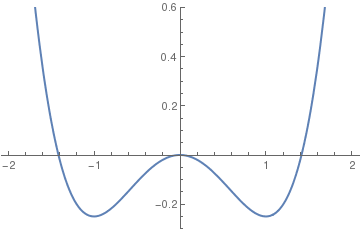We choose the units of length so that the minima are at $$x = \pm 1 ,$$ and the units of energy so that the depth of each well is at -1/4. The potential is given by $$V(x) = \frac{x^4}{4} - \frac{x^2}{2} .$$ The force is given by $$F(x) = - {\text d}V/{\text d}x = x- x^3 .$$ As usual we solve the second order differential equation F = ma by expressing it as two first order differential equations
$\frac{{\text d}x}{{\text d}t} =v, \qquad \frac{{\text d}v}{{\text d}t} = x- x^3$

where we set the mass equal to unity.

Because of energy conservation, one can clearly never get chaos from the motion of a single degree of freedom. We therefore add both a driving force and damping, in order to remove energy conservation. The equations of motion become

$\frac{{\text d}x}{{\text d}t} = v , \qquad \frac{{\text d}v}{{\text d}t} = x - x^3 - \gamma \,v + A\, \cos \left( \omega t \right) ,$
where γ is the friction coefficient, and A is the strength of the driving force which oscillates at a frequency ω. We will see that a transition to chaos now occurs as the strength of the driving force is increased. We will fix coefficients as $$\gamma = 0.05, \ \omega = 1.1 ,$$ and initially set A = 0.1 (which will be in the non-chaotic regime).

We now start the particle off at rest at the origin and integrate the equations of motion. We will go up to t = 200. In order to have sufficient accuracy we need to set the option MaxSteps of NDSolve to a larger value than the default (otherwise Mathematica will grumble).

sol = NDSolve[{v'[t] == x[t] - x[t]^3 - 0.05*v[t] + 0.1 Cos[1.1*t], x'[t] == v[t], x == 0, v == 0}, {x, v}, {t, 0, 200}, MaxSteps -> 3500];
We now show a phase space plot of the trajectory:
ParametricPlot[{x[t], v[t]} /. sol, {t, 0, 100}, AxesLabel -> {"x", "v"}]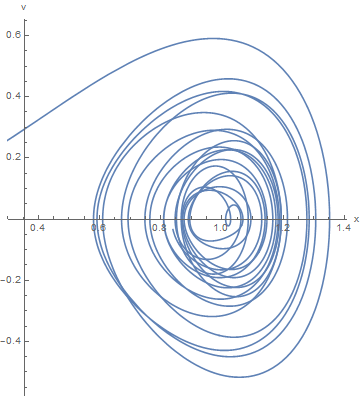This looks complicated, but in fact, most of the plot shows the initial period of time during which the motion is approaching its final behavior which is much simpler. The early behavior is called an "initial transient". One now sees a simple periodic orbit around the minimum of the potential at x = 1. We now investigate how the phase space plots change when the strength of the driving force is changed. It is convenient to define a function, which we call "solution[A, tmax]", which will integrate the equations for a given value of the forcing, A, up to a time tmax. We use a delayed assignment because we want the evaluation only to be done when we call the function later with particular values of A and tmax.
Clear[A];
solution[A_, tmax_] := NDSolve[{v'[t] == x[t] - x[t]^3 - 0.05*v[t] + A*Cos[1.1*t],
x'[t] == v[t], x == 0, v == 0}, {x, v}, {t, 0, tmax}, MaxSteps -> 100*tmax]
First we choose A = 0.2 and tmax = 800.
sol3 = solution[0.2, 800]
To plot the solution, it is convenient to first define a function
graph[tmin_, tmax_] := ParametricPlot[Evaluate[{x[t], v[t]} /. sol3],
{t, tmin, tmax}, AxesLabel -> {"x", "v"}]
graph[0, 200]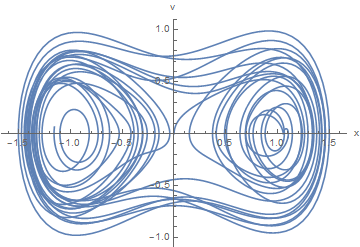Note that the particle has moved through both of the wells. However, again, most of this complexity is due to an initial transient. If we look at the behavior at later times, we take from 740 to 802, we see a much simpler curve:
graph[740, 802]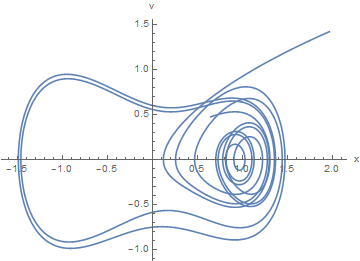Next let's increase the driving force to 0.338.
sol4 = solution[0.4, 1000]
graph[700, 800]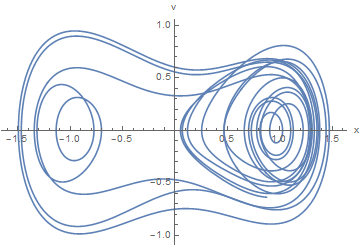Finally, we present animation of periodic change the velocity versus displacement of the chaotic attractor of the Duffing oscillator for β = 1, ω0² = 1, γ = 0.2, A = 0.3, and ω = 1.

A useful way of analyzing chaotic motion is to look at what is called the Poincaré section. Rather than considering the phase space trajectory for all times, which gives a continuous curve, the Poincaré section is just the discrete set of phase space points of the particle at every period of the driving force, i.e. at $$t= 2\pi /\omega , \ 2\pi /\omega , 3\pi /\omega , \ldots ,$$ etc. Clearly for a periodic orbit the Poincaré section is a single point, when the period has doubled it consists of two points, and so on.

We define a function, poincare, which produces a Poincaré section for given values of A, γ, and ω, in which the first ndrop periods are assumed to be initial transient and so are not plotted, while the subsequent ndrop eriods are plotted. The point size is given by the parameter psize. Note that the function g[{xold, vold}] maps a point in phase space {xold, vold} at time t to the point in phase space {x , v} one period T later

poincare[A_, gamma_, omega_, ndrop_, nplot_, psize_] := (T = 2*Pi/omega ;
g[{xold_, vold_}] := {x[T], v[T]} /. NDSolve[{v'[t] == x[t] - x[t]^3 - gamma*v[t] + A*Cos[omega*t],
x'[t] == v[t], x == xold, v == vold}, {x, v}, {t, 0, T}][];
lp = ListPlot[Drop[NestList[g, {0, 0}, nplot + ndrop], ndrop], PlotStyle -> {PointSize[psize], Hue}, PlotRange -> All, AxesLabel -> {"x", "v"}])
If we put in parameters corresponding to a limit cycle of length 4 we get 4 points as expected.
poincare[0.338, 0.1, 1.4, 1900, 100, 0.02]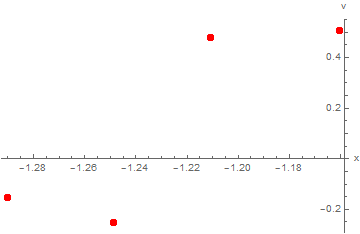However,the Poincaré section for parameters in the chaotic regime is much richer. Here we take A = 0.35:
poincare[0.35, 0.1, 1.4, 200, 15000, 0.002];
Show[lp, Epilog -> {GrayLevel[0.6], Thickness[0.006],
Line[{{0.8, 0.35}, {0.8, 0.67}, {1.05, 0.67}, {1.05, 0.35}, {0.8, 0.35}}]}]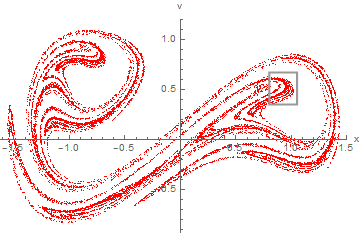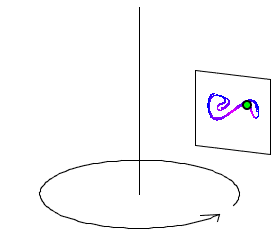This strange diagram is the strange attractor. It is the limiting set of points to which the trajectory tends (after the initial transient) every period of the driving force.

We now want to study the structure of the strange attractor in more detail. To do this we will blow up the region in the shaded box in the above figure. To do so, we define a function poincare2, in which we specify the range of x and v to be included in the plot:

poincare2[A_, gamma_, omega_, ndrop_, nplot_, psize_, xmin_, xmax_, vmin_, vmax_] := (T = 2*Pi/omega ;
g[{xold_, vold_}] := {x[T], v[T]} /. NDSolve[{v'[t] == x[t] - x[t]^3 - gamma*v[t] + A*Cos[omega*t], x'[t] == v[t], x == xold, v == vold}, {x, v}, {t, 0, T}][];
lp2 = ListPlot[Drop[NestList[g, {0, 0}, nplot + ndrop], ndrop], PlotRange -> {{xmin, xmax}, {vmin, vmax}},
PlotStyle -> {PointSize[psize], Hue}, PlotRange -> All, AxesLabel -> {"x", "v"}, AxesOrigin -> {xmin, vmin}, AspectRatio -> 1])
We zoom in to the region marked by the box in the figure above (this is quite slow because I had to increase the time of integration to 105 to get enough points in this region)
poincare2[0.35, 0.1, 1.4, 2000, 100000, 0.003, 0.8, 1.05, 0.35, 0.67];
The plot is then displayed including a box to indicate the region that will subsequently be blown up even more.
Show[lp2, Epilog -> {GrayLevel[0.6], Thickness[0.006],
Line[{{0.86, 0.48}, {0.91, 0.48}, {0.91, 0.58}, {0.86, 0.58}, {0.86, 0.48}}]}]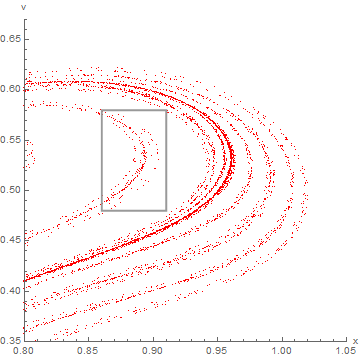Zooming in to the region in the box in the above figure, shows many of the same features as in the figure. (This takes a very long time, because I had to increase the length of the integration to 106 to get a significant density of points in this small region. You may want to skip the execution of the rest of this notebook, and just look at it.)
poincare2[0.35, 0.1, 1.4, 2000, 1000000, 0.005, 0.86, 0.91, 0.48, 0.58];
Show[lp2, Epilog -> {GrayLevel[0.6], Thickness[0.006],
Line[{{0.88, 0.525}, {0.88, 0.55}, {0.888, 0.55}, {0.888, 0.525}, {0.88, 0.525}}]}]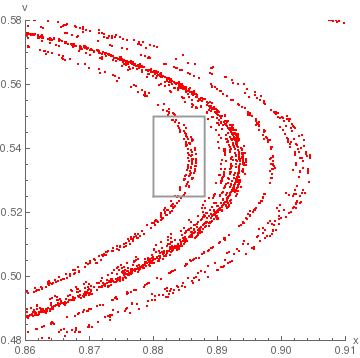Integrating a differential equation, as we have done here, is much more time consuming than iterating a map, such as the logistic map. People have therefore investigated maps which have similar behavior to that of driven, damped differential equations like the Duffing equation. One popular choice is the Hénon map:
$\begin{split} x_{n+1} &= 1 - a\,x_n^2 + y_n , \\ y_{n+1} &= b\,x_n , \end{split}$
in which two variables, x and y, are iterated. The parameters a and b can be adjusted to get a transition to chaos. In the chaotic regime, the points converge to a strange attractor similar to the one found for the Duffing equation. Note, in particular, the way it folds back on itself. A discussion of using Mathematica to display the Hénon map is given in
Zimmerman and Olness, Mathematica for Physics, Pearson, 2nd edition, 2002. ISBN-13: 978-0805387001

Going back to the Duffing equation, you can try different values of the parameters gamma and omega and see where the period doubling transition to chaos occurs. The function bifurcation below, is similar to poincare except that it scans a range of values of A, and gives the x-values on the attractor for each A.

bifurcation[dmin_ , dmax_ , nd_ , gamma_, omega_ , ndrop_, nplot_, psize_] := (T = 2*Pi/omega ;
g[{xold_, vold_}] := {x[T], v[T]} /. NDSolve[{v'[t] == x[t] - x[t]^3 - gamma*v[t] + A*Cos[omega*t],
x'[t] == v[t], x == xold, v == vold}, {x, v}, {t, 0, T}][];
f[{x_, y_}] := {A, x};
ListPlot[ Flatten[Table[ f /@ Drop[NestList[g, {1, 0}, nplot + ndrop], ndrop], {A, dmin, dmax, (dmax - dmin)/nd}], 1],
PlotStyle -> {PointSize[psize], Hue},
PlotRange -> {{dmin, dmax}, {-1.6, 1.6}}, AxesLabel -> {"A", "x"}, AxesOrigin -> {dmin, -1.6}])
The following command scans 200 values of A in the range from 0.3 to 0.35, with γ =0.1 and ω =1.4. It starts with v = 0 and x = 1, i.e.at rest in the x = 1 minimum. The equations are integrated for 1500 periods of the driving force with the first 1000 being ignored and the value of x plotted at the end of each of the remaining 500 periods.
bifurcation[0.30, 0.35, 200, 0.1, 1.4, 1000, 500, 0.006]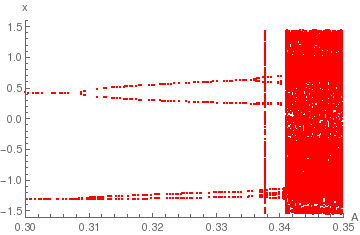One clearly sees period doubling leading into chaos. With the parameters chosen, in the region of limit cycles the system is either in the well at positive x or in the well at negative x, depending on the precise value of A, but does not hop between wells. For example, for A < 0.309 there are actually two separate fixed points rather than a period two limit cycle. (This accounts for the “spotty” nature of the lines, when one is blank the other is colored because the particle has converged on to one fixed point or the other.) However, in the chaotic region, the system goes between different wells.

bifurcation2, below, is similar to bifurcation, except that it also specifies the range of values of x (the vertical axis) that will be plotted.

bifurcation2[dmin_ , dmax_ , nd_ , xmin_, xmax_, gamma_, omega_ , ndrop_, nplot_, psize_] :=
(T = 2*Pi/omega ; g[{xold_, vold_}] := {x[T], v[T]} /.
NDSolve[{v'[t] == x[t] - x[t]^3 - gamma*v[t] + A*Cos[omega*t], x'[t] == v[t], x == xold, v == vold}, {x, v}, {t, 0, T}][];
f[{x_, y_}] := {A, x};
ListPlot[ Flatten[Table[ f /@ Drop[NestList[g, {1, 0}, nplot + ndrop], ndrop], {A, dmin, dmax, (dmax - dmin)/nd}], 1], PlotStyle -> {PointSize[psize], Hue}, PlotRange -> {{dmin, dmax}, {xmin, xmax}}, AxesLabel -> {"A", "x"}])
bifurcation2[0.334, 0.342, 200, -1.5, -1, 0.1, 1.4, 3500, 1000, 0.006]1. Armitage, J.V. and Eberlein, W.F., Elliptic Functions, Cambridge University Press, 2006.
2. Brennan, M.J.; Kovacic, I.; Carrella, A.; Waters, T.P. On the jump-up and jump-down frequencies of the Duffing oscillator, Journal of Sound and Vibration, 2008, Vol. 318 (4–5): 1250–1261. doi:10.1016/j.jsv.2008.04.032
3. Byrd, P.F. and Friedman, M.D., Handbook of Elliptic Integrals for Engineers and Scientists, Springer, 1971.
4. Duffing, G. (1918), Erzwungene Schwingungen bei veränderlicher Eigenfrequenz und ihre technische Bedeutung [Forced oscillations with variable natural frequency and their technical relevance; PhD Thesis] (in German), Heft 41/42, Braunschweig: Vieweg.
5. J. Guckenheimer and P. Holmes, Nonlinear Oscillations, Dynamical Systems, and Bifurcations of Vector Fields, Springer-Verlag, 1983.
6. Holmes, P.J. and Rand, D.A., The bifurcations of Duffing's equation: An application of catastrophe theory, Journal of Sound and Vibration, 1976, 44, pp. 237--253.
7. Holmes, P., A nonlinear oscillator with a strange attractor, Philosophical Transactions of the Royal Society A, 292, 419-448, 1979.
8. Holmes, C. and Holmes, P., Second order averaging and bifurcations to subharmonics in Duffing's equation, Journal of Sound and Vibration, 1981, 78, pp. 161--174.
9. Holmes P. and Whitley, D., On the attracting set for Duffing's equation, II. A geometrical model for moderate force and damping, Physica, 1983, Vol. 7D, pp. 111--123.
10. Kovacic, I. and Brennan, M.J., The Duffing Equation: Nonlinear Oscillators and their Behaviour, Wiley, 2011, ISBN: 978-0-470-97783-5
11. Milnor, J.W., (2006) Attractor. Scholarpedia, 1(11):1815.
12. Nayfeh, A. and Mook, D.T., Nonlinear Oscillations, Wiley-VCH, 1995. ISBN-13: 978-0471121428
13. Ott, E., Chaos in Dynamical Systems (2nd edition), Cambridge University Press, 2002.
14. Qin, Y., Zou, L., Wang, Z., Yu, Z., A Kind of Novel Analytical Approximate Solution to the Duffing Oscillator, Journal of Physics: Conference Series, Vol. 1168,
15. Rand, R.H., Lecture notes on nonlinear vibrations, 2012, version 53, Cornell University, Ithaca NY 14853, http://www.math.cornell.edu/∼rand/randdocs/
16. Salas, A.H. and Castillo, J.E., Exact Solution to Duffing Equation and the Pendulum Equation, Applied Mathematical Sciences, Vol. 8, 2014, no. 176, 8781 - 8789; http://dx.doi.org/10.12988/ams.2014.44243
17. Tajaddodianfar, F.; Yazdi, M.R.H.; Pishkenari, H.N. (2016). Nonlinear dynamics of MEMS/NEMS resonators: analytical solution by the homotopy analysis method. Microsystem Technologies, 2016, may; doi:10.1007/s00542-016-2947-7
18. J.M.T. Thompson and H.B. Stewart, Nonlinear Dynamics and Chaos (2nd edition), John Wiley & Sons, 2002.